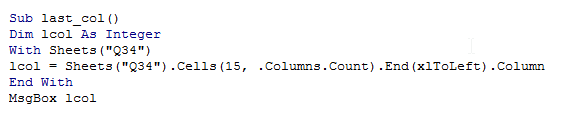## Vba last used column in Excel

In the previous chapter, we discussed about last used row.

This chapter deals with last used column in a worksheet. This also has its own importance in programming.

Similar to last used row, there might arise some occassions, where the new data has to be appended along a single row in multiple columns.

For this, the script should know about the last used column in a sheet.

This is where, this logic is used.

Unlike previous chapter, The last used column is found from Cells() object.

The Syntax is

1. lastColumn = Sheets().Cells(1, Sheets().Columns.Count).End(xlToLeft).Column

The same logic as before is used here.The excel traverses from very last column

until it finds a non-empty cell in row 1.

The following example illustrates this:

Note that, for demo purpose, we have put a value at Cell N15.

So we are going to find the last used column in Row 15, which is N(14th Column)

1. Sub last_col()
2. Dim lcol As Integer
3. With Sheets("Q34")
4. lcol = Sheets("Q34").Cells(15, .Columns.Count).End(xlToLeft).Column
5. End With
6. MsgBox lcol

The Screenshot of the editor is as shown below: